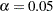Previous Page | Next Page

 The POWER Procedure

Example 68.8 Customizing Plots

This example demonstrates various ways you can modify and enhance plots:

• assigning analysis parameters to axes

• fine-tuning a sample size axis

• linking plot features to analysis parameters

• choosing key (legend) styles

• modifying symbol locations

The example plots are all based on a sample size analysis for a two-sampletest of group mean difference. You start by computing the sample size required to achieve a power of 0.9 by using a two-sided test with, assuming the first mean is 12, the second mean is either 15 or 18, and the standard deviation is either 7 or 9.

Use the TWOSAMPLEMEANS statement with the TEST=DIFF option to compute the required sample sizes. Indicate total sample size as the result parameter by supplying a missing value (.) with the NTOTAL= option. Use the GROUPMEANS=, STDDEV=, and POWER= options to specify values of the other parameters. The following statements perform the sample size computations:

```proc power;
twosamplemeans test=diff
groupmeans   = 12 | 15 18
stddev       = 7 9
power        = 0.9
ntotal       = .;
run;
```

Default values for the NULLDIFF=, SIDES=, GROUPWEIGHTS=, and DIST= options specify a null mean difference of 0, two-sided test, balanced design, and assumption of normally distributed data, respectively.

Output 68.8.1 shows that the required sample size ranges from 60 to 382, depending on the unknown standard deviation and second mean.

Output 68.8.1 Computed Sample Sizes
The POWER Procedure
Two-sample t Test for Mean Difference

Fixed Scenario Elements
Distribution Normal
Method Exact
Group 1 Mean 12
Nominal Power 0.9
Number of Sides 2
Null Difference 0
Alpha 0.05
Group 1 Weight 1
Group 2 Weight 1

Computed N Total
Index Mean2 Std Dev Actual Power N Total
1 15 7 0.902 232
2 15 9 0.901 382
3 18 7 0.904 60
4 18 9 0.904 98Previous Page | Next Page | Top of Page